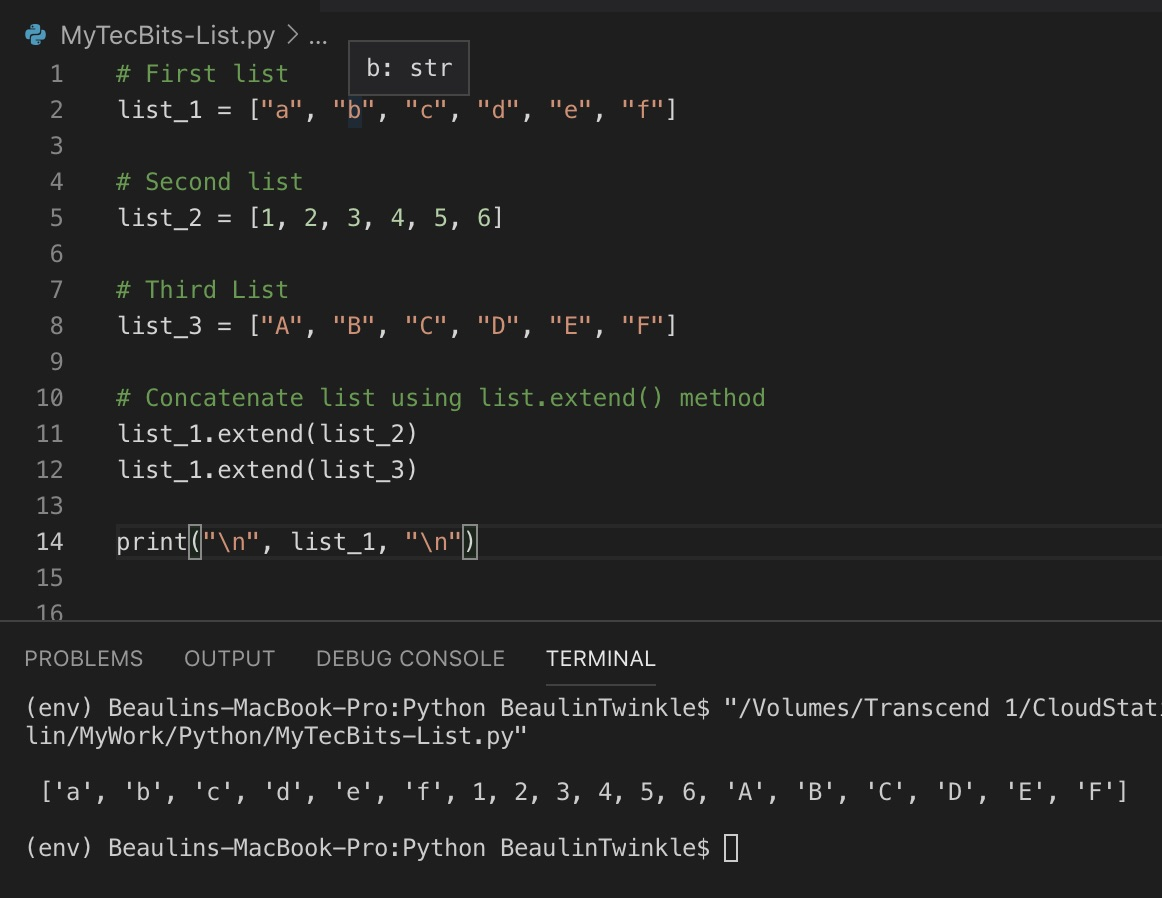# How to concatenate multiple lists in Python?

There are couple of efficient ways to merge / join / concatenate multiple lists in Python programming. They have their own advantages. Let’s see how to use them with example.

## Using + operator

One of the commonly used practice to concatenate two lists is to use the + operator. Using this method, you can even join more than two lists at the same time. The + operator add the elements of multiple lists and assign the result to another list. Here is an example.

### Example using + operator

```# First list
list_1 = ["a", "b", "c", "d", "e", "f"]

# Second list
list_2 = [1, 2, 3, 4, 5, 6]

# Third List
list_3 = ["A", "B", "C", "D", "E", "F"]

# Concatenate list using + operator
list_result = list_1 + list_2 + list_3

print(list_result)
```

#### Result

```['a', 'b', 'c', 'd', 'e', 'f', 1, 2, 3, 4, 5, 6, 'A', 'B', 'C', 'D', 'E', 'F']
```

## Using list.extend() method

Another practice to concatenate two lists is to use the list.extend() method. Using this method, you can merge or merge only two lists at the same time. For joining more then two lists, you have to use this method multiple times. Unlike + operator, list.extend() extends a list by appending the elements of another list at the end. Let us see an example of using list.extend().

### Example using list.extend() method

```# First list
list_1 = ["a", "b", "c", "d", "e", "f"]

# Second list
list_2 = [1, 2, 3, 4, 5, 6]

# Third List
list_3 = ["A", "B", "C", "D", "E", "F"]

# Concatenate list using list.extend() method
list_1.extend(list_2)
list_1.extend(list_3)

print(list_1)
```

#### Result

```['a', 'b', 'c', 'd', 'e', 'f', 1, 2, 3, 4, 5, 6, 'A', 'B', 'C', 'D', 'E', 'F']
```

## Reference

### Related Articles & Tools

This site uses Akismet to reduce spam. Learn how your comment data is processed.